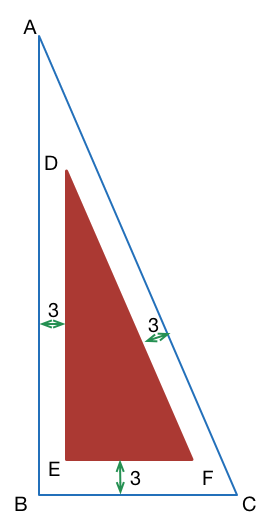Geometry Level 5Let $\Delta ABC$ have integer side lengths and be right-angled at $B$. Now suppose $\Delta DEF$ is located within $\Delta ABC$ with the same orientation, such that its sides are parallel to the corresponding sides of $\Delta ABC$ and the distance between the corresponding parallel sides is $3$.

If the area of $\Delta ABC$ is $4$ times that of $\Delta DEF$ then there are $n$ possible triangles $\Delta ABC$ independent of orientation. If $S$ is the sum of the lengths of the hypotenuses of these $n$ triangles, then find $n + S.$

×